Next: Isothermal and Adiabatic Expansion Up: Classical Thermodynamics Previous: Specific Heat

# Calculation of Specific Heats

Now that we know the relationship between the molar specific heats at constant volume and constant pressure for an ideal gas, it would be interesting if we could calculate either one of these quantities from first principles. Classical thermodynamics cannot help us here. However, it is straightforward to calculate the specific heat at constant volume using our knowledge of statistical physics. Recall that the variation of the number of accessible states of an ideal gas with energy and volume is written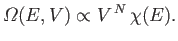(6.42)

In fact, for the specific case of a monatomic ideal gas, we worked out a more exact expression for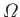in Section 3.8: namely,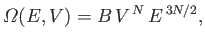(6.43)

where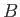is a constant independent of the energy and volume. It follows that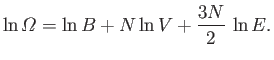(6.44)

The absolute temperature is given by [see Equation (5.30)]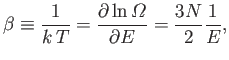(6.45)

so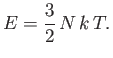(6.46)

Because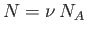, and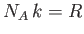, we can rewrite the previous expression as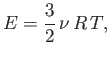(6.47)

where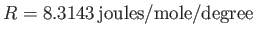is the molar ideal gas constant. The previous formula tells us exactly how the internal energy of a monatomic ideal gas depends on its temperature.

The molar specific heat at constant volume of a monatomic ideal gas is clearly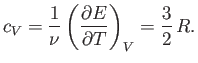(6.48)

This has the numerical value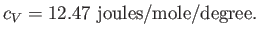(6.49)

Furthermore, we have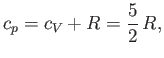(6.50)

and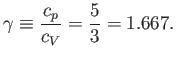(6.51)

We can see from Table 6.1 that these predictions are borne out fairly well for the monatomic gases helium and argon. Note that the specific heats of polyatomic gases are larger than those of monatomic gases, because polyatomic molecules can rotate around their centers of mass, as well as translate, so polyatomic gases can store energy in the rotational, as well as the translational, energy states of their constituent particles. We shall analyze this effect in greater detail later on in this course. (See Section 7.13.)Next: Isothermal and Adiabatic Expansion Up: Classical Thermodynamics Previous: Specific Heat
Richard Fitzpatrick 2016-01-25